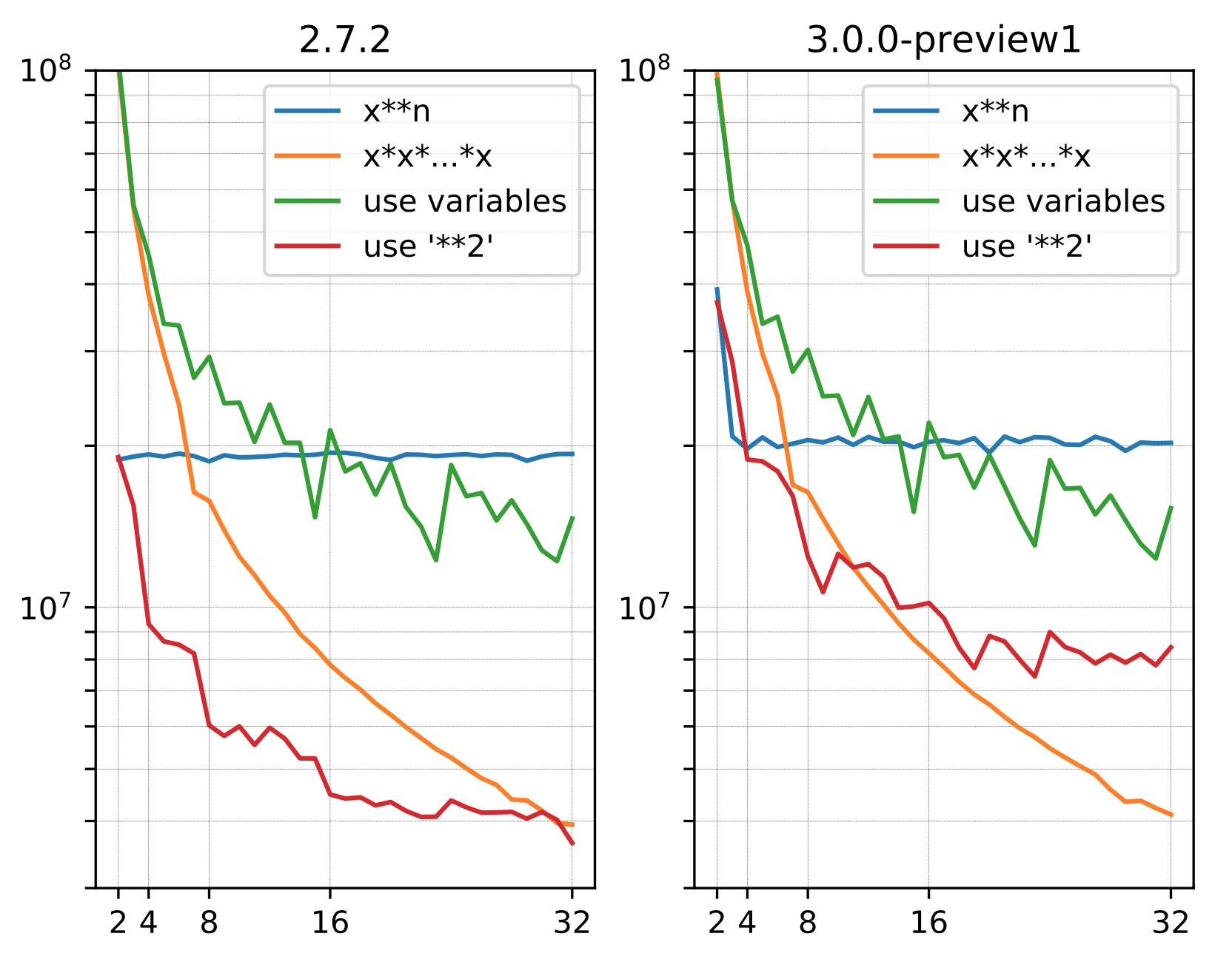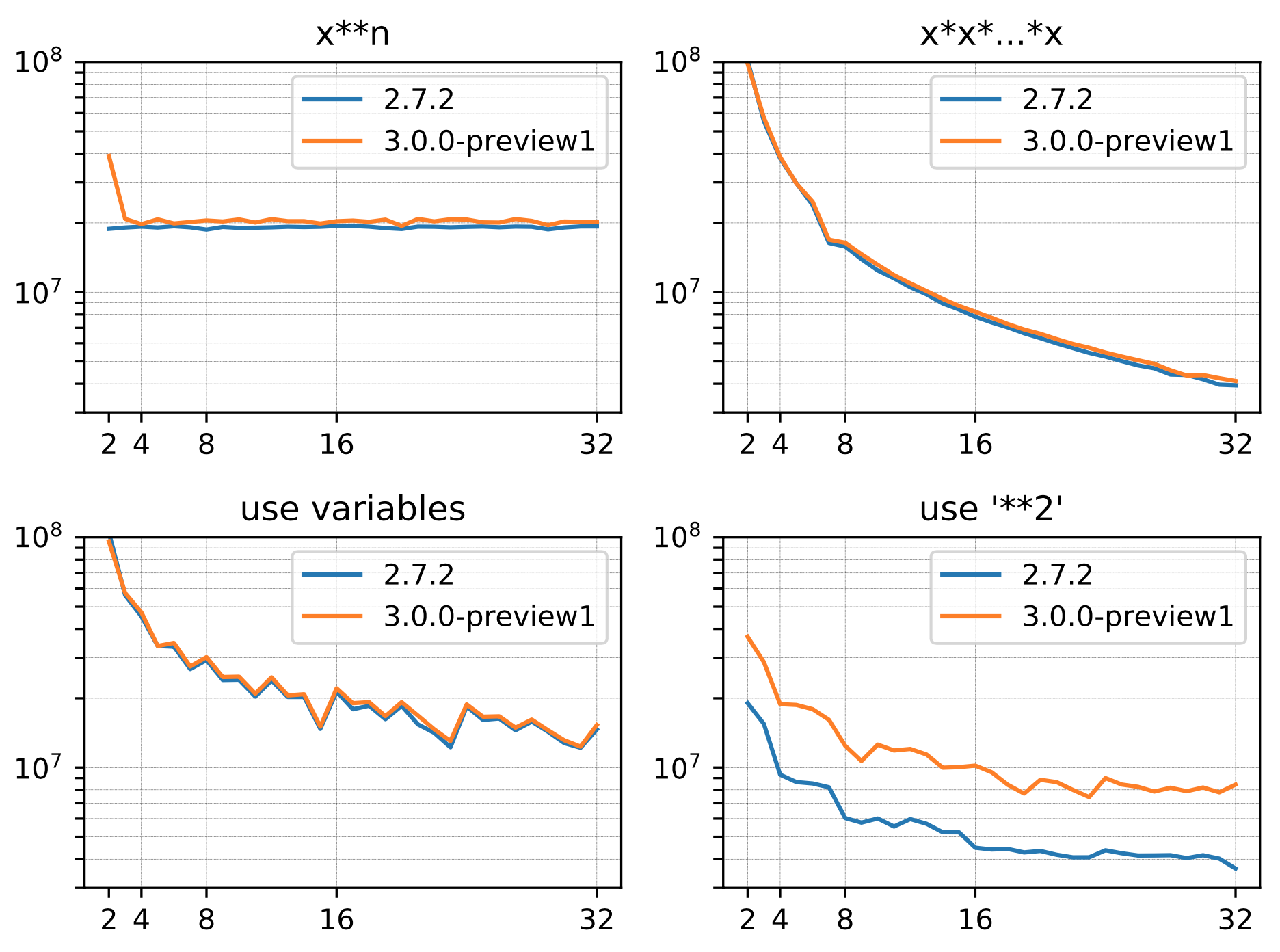# What is this?

After reading Ruby 3.0 makes x ** 2 faster, I wanted to microbenchmark, so I microbenchmarked.

It doesn't stand from the viewpoint of practicality.

# What to measure

ruby is 2.7.2 and 3.0.0-preview1. The environment is macOS Catalina 10.15.6. 2.2 GHz quad core Intel Core i7.

# What to measure

For example, suppose you want to calculate the 11th power of `x`. There are the following methods.

name Method of calculation
`x**n` `x**11`
`x*x*...*x` `x*x*x*x*x*x*x*x*x*x*x`
`use variables` `z=x*x;y=x*z*z;x*y*y`
`use '**2'` `((x**2)**2*x)**2*x`

The first two are good. ʻUse variables` makes a decent amount of effort to reduce the number of multiplications. I didn't make every effort to reduce it, so for example, in the case of 27, "` z = x * x * x; y = z * z; z = x * y * y; x * z * z` "7 times It has become. If I try my best, it will probably be "` z = x * x * x; y = z * z * z; y * y * y` "6 times, but I skipped it.

The last one is calculated using a lot of `** 2`.

x is set to `3.0`. I tried the index from 2 to 32. If the exponent value is small, `x ** n` and ʻuse'** 2'` are the same, and` x * x * ... * x` and ʻuse variables` are also the same.

# result

All the graphs are Iteration per second, so the top is fast. Note the semi-log graph.ʻUse variables` seems to be fast up to the 10th power. Up to about 16th power, ʻuse variables` and` x ** n` are pulled out. More than that, `x ** n` is faster.

Looking at the graph, it seems better to treat up to `x ** 3` or` x ** 4` specially, but what about it?If you look at `x ** n`, you can see that 3.0.0-preview1 treats` ** 2` specially.